# De-trending climate data¶

For some projects, we need to use the projected climate scenarios without the warming (or cooling) trend that is present in each month (or season).

For instance in a situation where, over 100 years, January becomes warmer, but July becomes cooler, we would like to remove the warming trend from January and the cooling trend from July.

We'd like to remove any trend that might be present for a given month over the next 100 years, for every pixel in the AIEM domain.

A single climate driver variable for dvmdostem, over the whole AIEM domain for 100 years at 1km spatial resolution and 1 monthly time resolution takes ~21GB per variable:

1850*2560 = 4,736,000 pixels in AIEM domain, including oceans, etc.

4 bytes
----------- * 12 months * 200 years * (1850*2560) = 22,732,800,000 bytes
32bit float

22,732,800,000 / 1024 / 1024 / 1024 = 21.17 GB



We need to do this for 4 variables (air temp, vapor pressure, near infrared radiation, and precipitation) for six scenarios (echam, hadley? ccma? etc?). Thus we need to process ~500GB of data.

In this notebook we will outline the process for removing a trend from the timeseries climate data downloaded from SNAP in a (hopefully) computationally reasonable amount of time: (??)

## Setup¶

### Software¶

This notebook will call some external shell commands, so you must have the appropriate software installed and available on the path that IPython uses. For the most part things should work if you install your software with the system package manager (e.g. apt-get or yum or homebrew)

• GDAL command line utilities (specifically gdal_translate)

Also you will need the following python libraries.

• netCDF4
• matplotlib
• scipy.signal
• rasterio
• IPython Notebook (for running or adding to this .ipynb file)

### Data¶

The code in this notebook assumeses that you have downloaded the climate files from SNAP and have extracted all the .tifs so that you have a directory strucutre something like this (assuming you are working with temperature). Note that the data is organized with a .tif image for each month for the next 100 years (1200 files):

├── tas_mean_C_iem_cccma_cgcm3_1_sresa1b_2001_2100
│   ├── tas_mean_C_iem_cccma_cgcm3_1_sresa1b_01_2001.tif
│   ├── tas_mean_C_iem_cccma_cgcm3_1_sresa1b_01_2002.tif
|   ........
│   ├── tas_mean_C_iem_cccma_cgcm3_1_sresa1b_12_2099.tif
│   └── tas_mean_C_iem_cccma_cgcm3_1_sresa1b_12_2100.tif

For this notebook, we are working in a directory

## Initial Exploration¶

There are many techniques for de-trending data. Ideally we can use scipy.signal.detrend from SciPy's signal processing library and avoid having to devise our own custom detrending algorithm.

So first we need to play around with scipy.signal.detrend until we are convinced it will work for our needs.

In :
import numpy as np
import matplotlib.pyplot as plt
import scipy.signal

# IPython "magic" to allow plots to be displayed inline
%matplotlib inline


Next, create an x range and a few basic signals over that range:

In :
SIZE = 1000
x = np.arange(0, SIZE)

noise = np.random.uniform(low=-50, high=50, size=SIZE)
sin_wave = 100 * np.sin( (np.pi/180) * x )
trend = np.linspace(-500, 500, SIZE)


Checkout what we have created so far:

In :
plt.plot(x, noise, label='noise')
plt.plot(x, sin_wave, label='sin wave')
plt.plot(x, trend, label = 'trend')
plt.legend()

Out:
<matplotlib.legend.Legend at 0x18adf9cd0>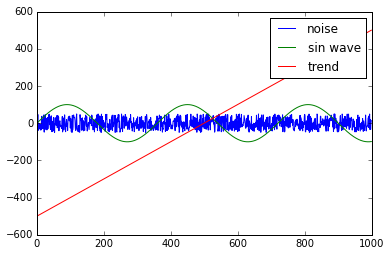Now we can add our basic components together to create a noisy, trending, sin wave. This should be somewhat similar to how one of our climate variables might look:

In :
noisy_sin = noise + sin_wave
noisy_trending_sin = noisy_sin + trend

plt.plot(x, noisy_sin, label='noisy sin wave')
plt.plot(x, noisy_trending_sin, label='noisy, trending, sin wave')
plt.legend()

Out:
<matplotlib.legend.Legend at 0x18ab36850>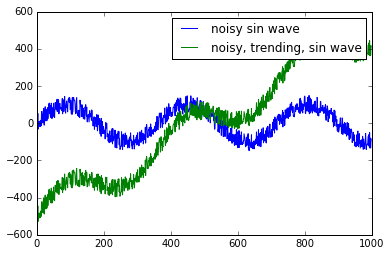Looking pretty good. Now to see what exactly the scipy.signal.detrend function does. There are two options for how you'd like the detrending to happen, 'linear' and 'constant'. Accodring to the documentation, the linear subtracts the result of a least-squares fit for the the signal from the signal. The constant option subtracts the mean of the signal from the original signal.

In :
lin_detrend = scipy.signal.detrend(noisy_trending_sin, type='linear')
con_detrend = scipy.signal.detrend(noisy_trending_sin, type='constant')

plt.plot(x, noisy_trending_sin, label='noisy trending sin')
plt.plot(x, lin_detrend, label='linear detrend')
plt.plot(x, scipy.signal.detrend(noisy_trending_sin, type='constant'), label='constant detrend')

plt.legend()

Out:
<matplotlib.legend.Legend at 0x18ab6f3d0>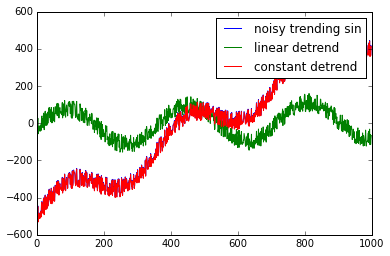Huh, interesting. Looks like the linear option is what we want for our application.

However, it looks like the linear detrending seems to center the resulting signal around zero, so we will need to offset the result to line up with the initial reading in the initial signal:

In :
offset_lin_detrend = lin_detrend + (noisy_trending_sin - lin_detrend)
plt.plot(x, noisy_trending_sin, label='noisy trending sin')
plt.plot(x, offset_lin_detrend,  label='offset linear detrend')
plt.legend()

Out:
<matplotlib.legend.Legend at 0x18b26ef10>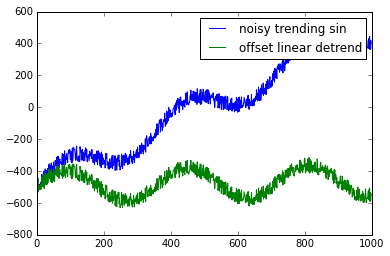So that is looking pretty darn good. If we end up needing a more complicated line fitting algorithm (e.g. cubic spline) for finding the trend maybe we can riff on the scipy.signal.detrend.

For now this seems like it should work. Lets try it on some of our actual data. But first, lets cleanup and release the memory needed for this test:

In :
%reset -f


## Try with real data¶

First we need to load up some libraries for working with the .tif files from SNAP. After the reset magic from above, we have to grab numpy, matplotlib and scipy.signal again too:

In :
import numpy as np
import matplotlib.pyplot as plt
import scipy.signal

import rasterio
import netCDF4  # Not using this yet, but may want to write netcdf file??


Next we will use GDAL's notion of "Virtual Raster Tiles" to assemble a "view"of our 1200 files that shows only one month, but for the entire set of years. We are essentially creating a new file by combining all the files that would be listed with this command:

\$ ls tas_mean_C_iem_cccma_cgcm3_1_sresa1b_01_*.tif



So, 100 files; 100 years worth of January.

GDAL Utilities has a tool, gdalbuildvrt, that can quickly create such a "view file", or "Virtual Raster Tile" file that behaves just like a .tif would. This is essentially an "index" into a set of .tif input files, and allows us to access the data in the "shape" we want without having to create a copy of the data. The "Virtual Raster Tile" file has extension .vrt. Beacause a .vrt file behaves identically to a .tif, we can open and handle the .vrt files with the same programs or tools we might use on a tif file, but the .vrt file can be composed from a subset of one or more bands in one or more input files.

In our case we want to be able to open the .vrt file and read it into a numpy datastructure so that we can use signal.scipy.detrend on the data; we will likely want to write this detrended numpy datastructure out to a new file as well.

We will use the exclamation mark to access the system shell commands for ls and gdalbuildvrt. We will also use the IPyhton magic to "time" the execution, and "reset" to free up some memory.

In :
# Create a list of the files we'd like to include
# in our virtual dataset. For starters, we'll
# just pick January for all years:
!ls ../../snap-data/tas_mean_C_iem_cccma_cgcm3_1_sresa1b_2001_2100/tas_mean_C_iem_cccma_cgcm3_1_sresa1b_01* > janfiles.txt

# Next, we ask gdalbuildvrt to create a .vrt file
# from all the files in the list we just created.
#   -seperate      each file to a stacked band
#   -b 1           use band number 1 from each input file
%time !gdalbuildvrt -separate -b 1 -input_file_list janfiles.txt jan.vrt

0...10...20...30...40...50...60...70...80...90...100 - done.
gdalbuildvrt(5952,0x7fff7f226310) malloc: *** error for object 0x7fbd88f0add0: pointer being freed was not allocated
*** set a breakpoint in malloc_error_break to debug
CPU times: user 6.58 ms, sys: 6.77 ms, total: 13.4 ms
Wall time: 770 ms


Not too bad for creating an index into ~20GBs of (sorted) data.

NOTE: Not sure what the malloc error in gdalbuildvrt is all about. Seems to come up after the operation is "done" though, so it seems like our file should be ok. Maybe we should report a bug.

Now we can open and read the .vrt file just as we would a .tif file:

In :
month_temperature_file = rasterio.open("jan.vrt")

In :
# this one takes a while...

CPU times: user 15 s, sys: 1.16 s, total: 16.2 s
Wall time: 16.2 s


This is for one month, so to do all months, we are looking at (~15secs * 12months)/(60secs/min) ~= 3, so about three minutes of file-reading time for one variable, one scenario. ~3 min x 4 variables x 6 scenarios comes out to ~72 minutes of file-reading time for all our data. Not trivial, but totally reasonable for nearly 500GBs of data...

If we look at the data we can see that it looks to be the correct shape. The axes (dimensions) appear to be (time, y, x). So we have a "stack" of images, with each item in the stack being a point along the time axis:

In :
# dimensions are (time, y, x)
# from upper left corner of image
# (not sure which corner of the pixel)

print "Type of data: ", type(temperature_data)
print "Size of data: ", temperature_data.nbytes/1024/1024, "(Mbs)"
print "Shape of data: ", temperature_data.shape
print "Min value: ", np.min(temperature_data)
print "Max value: ", np.max(temperature_data)

Type of data:  <class 'numpy.ma.core.MaskedArray'>
Size of data:  1806 (Mbs)
Shape of data:  (100, 1850, 2560)
Min value:  -3.4e+38
Max value:  11.5998


Not sure why, but it seems that even though our data is a masked array and the fill value seems correct, the mask doesn't seem to be used because the upper left corner pixel, which should be masked out, shows up:

In :


In :
print "Using mask?:", temperature_data.mask
print "Fill value:", temperature_data.fill_value
print "First value (should be masked):", temperature_data[0,0,0]

Using mask?: False
Fill value: -3.4e+38
First value (should be masked): -3.4e+38


If we don't ensure that the oceans and other no-data areas are recoginized and appropriately excluded from calculations and displays, then the automatic scale-ing can make plots difficult to interpert. Usually the scale ends up far too large in order to accomodate the very-large or very-small values used for no-data pixels.

In :
temperature_data = np.ma.masked_outside(temperature_data, -500, 500, copy=False)


Now if we take a look at the value for the first time-step (first image in the stack), upper left pixel, we see it is masked, which makes sense in light of the image:

In :
print "First value: (should be masked):", temperature_data[0,0,0]
print ""
plt.imshow(temperature_data[0,:,:])
plt.colorbar()

First value: (should be masked): --


Out:
<matplotlib.colorbar.Colorbar instance at 0x10add1128>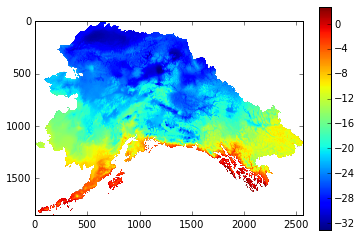In :
# Next, we can look at the timeseries for a
# pixel in central alaska that should have data
plt.plot(temperature_data[:, 550, 1050])

Out:
[<matplotlib.lines.Line2D at 0x10a846dd0>]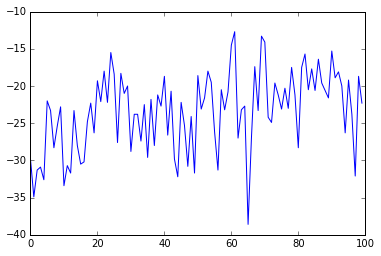Now we can run the scipy.signal.detrend function over the time axis for every pixel on the map:

In :
# Another 15 seconds or so and a lot of memory. There might
# be a better way to do this.
%time dtrd = scipy.signal.detrend(temperature_data, axis=0)

CPU times: user 12.4 s, sys: 4.17 s, total: 16.6 s
Wall time: 13.3 s

/Users/tobeycarman/.pyenv/versions/ipy-notebook/lib/python2.7/site-packages/scipy/linalg/basic.py:552: RuntimeWarning: overflow encountered in square
resids = np.sum(np.abs(x[n:])**2, axis=0)


And then look at the detrended timeseries for a pixel. The scipy.signal.detrend centers the resulting signal around zero. We can offset the new timeseries signal so that it starts at the same value as the original timeseries by finding the difference between the first point in the original timeseries, and the first point in the detrended timeseries, and then adding that difference to the detrended series.

In :
Y = 550
X = 1050
plt.suptitle("Pixel(y,x): (%s, %s)" % (Y, X))
plt.plot(temperature_data[:,Y,X], label='original')
plt.plot(dtrd[:,Y,X], label='detrended')
plt.plot((dtrd[:,Y,X] + (temperature_data[0,Y,X] - dtrd[0,Y,X])), label='dtrend+offset')
plt.legend()

Out:
<matplotlib.legend.Legend at 0x10a8a71d0>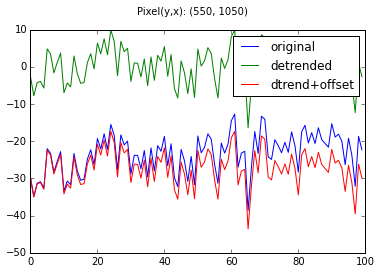It turns out it is blazingly fast to add the offset to the array in place:

In :
%time dtrd[:,:,:] += (temperature_data[0,:,:] - dtrd[0,:,:])

CPU times: user 295 ms, sys: 4.46 ms, total: 300 ms
Wall time: 300 ms

-c:1: RuntimeWarning: overflow encountered in add


Now the timeseries for each pixel in the image has been detrended and offset so that the detrended timeseries begins at the same value as the original timeseries.

In :


In :
def check_pixel(orig, detrended, Y = 550, X = 1050):
plt.suptitle("Pixel(y,x): (%s, %s)" % (Y, X))
plt.plot(orig[:,Y,X], label='original')
plt.plot(detrended[:,Y,X], label='detrended')
plt.legend()

In :
check_pixel(temperature_data, dtrd, Y=550,X=1050)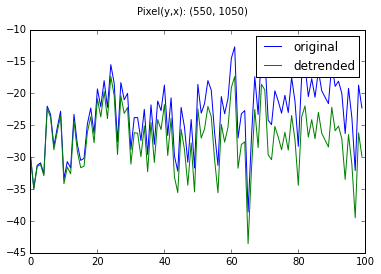In :
check_pixel(temperature_data, dtrd, Y=1200, X=780)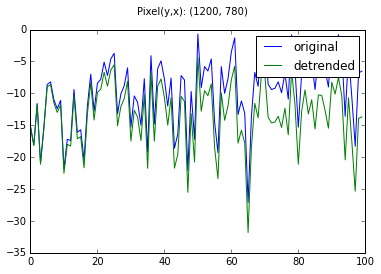Now that we have the method worked out we will write a more generalized script that can be used on all four variables and likely will concatenate the months to create new output series in the same shape/format as the original input tifs.

In :
# Yikes, lots of memory use!
# See what we are using:
print dtrd.nbytes/1024.0/1024.0/1024.0, "GBs"
print temperature_data.nbytes/1024.0/1024.0/1024.0, "GBs"

1.76429748535 GBs
1.76429748535 GBs

In :


In :


In :


In :
%reset -f

In :


In :
import numpy as np
import matplotlib.pyplot as plt
import rasterio
ds_orig = rasterio.open('orig.vrt', 'r')
ds_new = rasterio.open('month-12-new.vrt', 'r')
plt.imshow(ds_orig_data)
plt.ion()
plt.show()
ds_orig_data = np.ma.masked_outside(ds_orig_data, -500, 500, copy=False)
ds_new_data = np.ma.masked_outside(ds_new_data, -500, 500, copy=False)
plt.imshow(ds_orig_data)
f0 = plt.figure()
f1 = plt.figure()
plt.plot(ds_new_data[0,550,1050])
plt.plot(ds_new_data[:,550, 1050])
plt.plot(ds_orig_data[:,550,1050], label='orig')
plt.plot(ds_new_data[:,550,1050], label='detrended')
ds_orig_data[0,0,0]
ds_orig_data[0,550,1050]
ds_new_data[0,550,1050]
plt.plot(ds_new_data[:,551,1050], label='detrended')
plt.plot(ds_orig_data[:,551,1050], label='orig')
plt.plot(scipy.signal.detrend(ds_orig_data[:,551,1050], label='fresh detrend')
)
import scipy.signal
plt.plot(scipy.signal.detrend(ds_orig_data[:,551,1050], label='fresh detrend')
)
plt.plot(scipy.signal.detrend(ds_orig_data[:,551,1050], axis=0), label='fresh detrend')
)
plt.plot(scipy.signal.detrend(ds_orig_data[:,551,1050], axis=0), label='fresh detrend')
fdt = scipy.signal.detrend(ds_orig_data[:,551,1050], axis=0
)
fdt
ds_orig_data[0,551,1050]
ds_new_data[0,551,1050]
fdt+=ds_orig_data[0,551,1051]
fdt-=ds_orig_data[0,551,1051]
fdt + ds_orig_data[0,551,1051]
plt.plot(fdt + ds_orig_data[0,551,1050], label='fresh detrend + offset')
plt.legend()
plt.plot(fdt + (ds_orig_data[0,551,1050]-fdt), label='fresh detrend + offset2')
plt.legend()
history

In :


In :


In :


In :


In :


In :


In :


In :


In :


In :


In :


In :


In :


In :


In :


In :


In :


In :


In :


In :


In :


In :


In :


In [ ]: